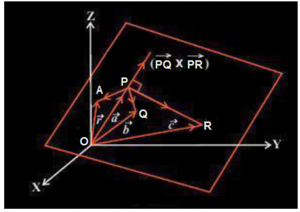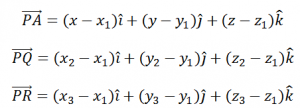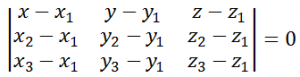Checkout JEE MAINS 2022 Question Paper Analysis : Checkout JEE MAINS 2022 Question Paper Analysis :

# Equation Of A Plane: 3 Non-Collinear Points

The physical quantities which have magnitude, as well as directly attached to them, are known as vectors. Position vectors simply denote the position or location of a point in the three dimensional Cartesian systems with respect to a reference origin. In the upcoming discussion, we shall study about vectors and the Cartesian equation of a plane in three dimensions.

In the previous section we have already discussed that to a given vector there lie infinite planes which are perpendicular to it. But for a particular point there exist one and only one unique plane which is perpendicular to it passing through the given point. The vector equation of a plane in this case is given as

$$\begin{array}{l}(\vec{r} – \vec{a}) . \vec{N} = 0\end{array}$$

Here,

$$\begin{array}{l}\vec{r} \end{array}$$
and
$$\begin{array}{l}\vec{a} \end{array}$$
represent the position vectors.

The Cartesian equation of such a plane is represented by:

$$\begin{array}{l}A(x-{x}_{1}) + B (y- {y}_{1}) + C (z -{z}_{1}) = 0 \end{array}$$

Here, A, B, and C are the direction ratios.

Let us now discuss the equation of a plane passing through three points which are non-collinear.

## Equation of a Plane Passing through Three Non Collinear Points

Let us consider three non collinear points P, Q, R lying on a plane such that their position vectors are given by

$$\begin{array}{l}\vec{a} \end{array}$$
,
$$\begin{array}{l}\vec{b} \end{array}$$
and
$$\begin{array}{l}\vec{c} \end{array}$$
as shown in the figure given below.The vectors

$$\begin{array}{l}\vec{PQ} \end{array}$$
and
$$\begin{array}{l}\vec{PR} \end{array}$$
lie in the same plane. The vector lying perpendicular to plane containing the points P, Q and R is given by
$$\begin{array}{l}\vec{PQ} \end{array}$$
×
$$\begin{array}{l}\vec{PR} \end{array}$$
. If  is the position vector of any point A lying in the plane containing P, Q, R then using the vector equation of a plane as mentioned above, the equation of the plane passing through P and perpendicular to the vector
$$\begin{array}{l}\vec{PQ} \end{array}$$
×
$$\begin{array}{l}\vec{PR} \end{array}$$
is given by

(

$$\begin{array}{l}\vec{r} \end{array}$$
$$\begin{array}{l}\vec{a} \end{array}$$
). (
$$\begin{array}{l}\vec{PQ} \end{array}$$
×
$$\begin{array}{l}\vec{PR}) = 0\end{array}$$

Also, from the above figure,  and . Substituting these values in the above equation, we have

$$\begin{array}{l}(\vec{r} – \vec{a}).[(\vec{b}- \vec{a}) × (\vec{c}- \vec{a})] = 0 \end{array}$$

This represents the equation of a plane in vector form passing through three points which are non- collinear.

To convert this equation in Cartesian system, let us assume that the coordinates of the point P, Q and R are given as (x1, y1, z1), (x2, y2, z2) and (x3, y3, z3) respectively. Also let the coordinates of point A be  x, y and z.Substituting these values in the equation of a plane in Cartesian form passing through three non-collinear points, we haveThis is the equation of a plane in Cartesian form passing through three points which are non- collinear.

Stay tuned with BYJU’S – The Learning App and download the app to learn all Maths-related concepts quickly by exploring more videos.

## Frequently Asked Questions on Equation of a Plane Passing Through 3 Non-collinear Points

### What is meant by the equation of a plane?

The equation of a plane defines the plane surface in the three-dimensional space.

### Mention two different forms of the equation of a plane?

The equation of a plane can be expressed in two different forms such as vector form and cartesian form.

### What are the different methods to derive the equation of a plane?

The equation of a plane can be derived using different methods, such as
Equation of a plane in normal form
Equation of a plane perpendicular to a given vector and through a point
Equation of a plane passing through three non-collinear points
Equation of a plane passing through the intersection of two given planes.

### Is one normal and one point sufficient to form the equation of a plane?

Yes, one normal and one point is sufficient to form the equation of a plane.

### What is the intercept form of the equation of a plane?

The intercept form of the equation of a plane is (x/a) +(y/b) +(z/c) =1. Here, a, b and c are the x-intercept, y-intercept and z-intercept respectively.